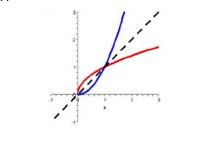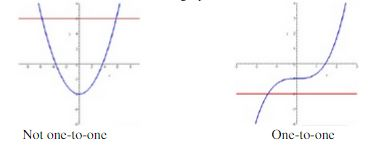Ontario Tech acknowledges the lands and people of the Mississaugas of Scugog Island First Nation.

We are thankful to be welcome on these lands in friendship. The lands we are situated on are covered by the Williams Treaties and are the traditional territory of the Mississaugas, a branch of the greater Anishinaabeg Nation, including Algonquin, Ojibway, Odawa and Pottawatomi. These lands remain home to many Indigenous nations and peoples.

We acknowledge this land out of respect for the Indigenous nations who have cared for Turtle Island, also called North America, from before the arrival of settler peoples until this day. Most importantly, we acknowledge that the history of these lands has been tainted by poor treatment and a lack of friendship with the First Nations who call them home.

This history is something we are all affected by because we are all treaty people in Canada. We all have a shared history to reflect on, and each of us is affected by this history in different ways. Our past defines our present, but if we move forward as friends and allies, then it does not have to define our future.

# Inverse Functions

The inverse of a function results when we have solved for x as the dependent variable and y as the independent variable and have renamed them according to the usual convention. For example, if f(x) gives the number of bacteria in a culture as a function of time (time is the independent variable and bacteria is the dependent variable), then f -1(x) gives the time it takes for the number of bacteria in the culture to reach a certain value (bacteria is now the independent variable, and time is the dependent variable). The inverse function is denoted as f -1(x) and is read "f inverse". Graphically, it's the mirror image of the given function around the line y = x.## Existence

For a function to have an inverse, the function must be one-to-one. This simply means that f can never take on the same value twice (i.e., that no two values produce the same f(x) value). The horizontal line test states that a function is one-to-one if and only if a horizontal line cannot intersect its graph more than once.## Domain and Range

The inverse function has a domain that is equivalent to the original function's range and a range that is equivalent to the original function's domain; therefore, if a function has a domain of A and a range of B, then the inverse function would have a domain of B and a range of A. This makes sense since we can think of an inverse as 'flipping' the function around the line y = x, so that all the x and y values get swapped.

## Finding an Inverse

To find the inverse of a one-to-one function, just follow the easy steps below:

• write the equation replacing f(x) with y;
• solve for x in terms of y;
• interchange x and y; and
• replace y with f -1(x).

In the above procedure, note that the order of the 2nd and 3rd steps can be interchanged.

One last thing to note about functions and their inverse is that the two cancel each other out. Therefore,
f(f -1(x)) = x and   f -1(f(x)) = x
These are "cancellation equations" since one function undoes what the other did.

Example: Find the inverse of f(x) = (x - 3) ÷ 4

Solution:

Rewrite using y instead of f(x): y = (x - 3) ÷ 4

Isolate for x:
4y = x - 3

4y + 3 = x

Switch x and y and replace y with f -1(x): f -1(x) = 4x +3

Example: Given f(x) = 9 ÷ (x - 2), find f -1(x) and its domain and range.

Solution:

Rewrite using y instead of f(x): y = 9 ÷ (x - 2)

Isolate for x:

y(x - 2) = 9

x - 2 = 9/y

x = 9/y  + 2

Switch x and y and replace with f -1(x): f -1(x)= 9/x  +  2

The domain of f(x) is x ≠ 2, x ∈ R. Since the domain of the original is the range of the inverse, the range of f -1(x) is y ≠ 2, y ∈ R. The range of f(x) is y ≠ 0, y ∈ R; therefore, the domain of f -1(x) is x ≠ 0, x ∈ R.

Example: